U.S. Department of Transportation
1200 New Jersey Avenue, SE
Washington, DC 20590
202-366-4000

Federal Highway Administration Research and Technology
Coordinating, Developing, and Delivering Highway Transportation InnovationsThis report is an archived publication and may contain dated technical, contact, and link information
 Federal Highway Administration > Publications > Research Publications > 05062 > Nondestructive Evaluation > Users Manual for LS-DYNA Concrete Material Model 159
Publication Number: FHWA-HRT-05-062
Date: May 2007

# Users Manual for LS-DYNA Concrete Material Model 159

PDF files can be viewed with the Acrobat® Reader®

### Foreword

This report documents a concrete material model that has been implemented into the dynamic finite element code, LS-DYNA, beginning with version 971. This model is in keyword format as MAT_CSCM for Continuous Surface Cap Model. This material model was developed to predict the dynamic performance-both elastic deformation and failure-of concrete used in roadside safety structures when involved in a collision with a motor vehicle. An example of a roadside safety structure is a concrete safety barrier that divides opposing lanes of traffic on a roadway. Default input parameters for concrete are stored in the model and can be accessed for use. This material model only replicates the concrete aggregate. Appropriate reinforcement bars or rods must be included in the structure model separately.

The Users Manual for LS-DYNA Concrete Material Model 159 is the first of two reports that completely document this material model. This report documents the theoretical basis, the required input format, and includes limited hypothetical problems for the user. The second report, Evaluation of LS-DYNA Concrete Material Model 159 (FHWA-HRT-05-063), documents the testing performed to document the model's performance and accuracy of results.

This report will be of interest to research engineers who are associated with the evaluation and crashworthy performance of roadside safety structures, particularly engineers responsible for predicting the crash response of such structures when using the finite element code, LS-DYNA.

Michael Trentacoste

Director, Office of Safety R&D

Notice

This document is disseminated under the sponsorship of the U.S. Department of Transportation in the interest of information exchange. The U.S. Government assumes no liability for the use of the information contained in this document.

The U.S. Government does not endorse products or manufacturers. Trademarks or manufacturers' names appear in this report only because they are considered essential to the objective of the document.

Quality Assurance Statement

The Federal Highway Administration (FHWA) provides high-quality information to serve Government, industry, and the public in a manner that promotes public understanding. Standards and policies are used to ensure and maximize the quality, objectivity, utility, and integrity of its information. FHWA periodically reviews quality issues and adjusts its programs and processes to ensure continuous quality improvement.

Technical Report Documentation Page

 1. Report No. FHWA-HRT-05-062 2. Government Accession No. 3. Recipient's Catalog No. 4. Title and Subtitle USERS MANUAL FOR LS-DYNA CONCRETE MATERIAL MODEL 159 5. Report Date May 2007 6. Performing Organization Code 7. Author(s) Yvonne D. Murray 8. Performing Organization Report No. 9. Performing Organization Name and Address APTEK, Inc. 1257 Lake Plaza Drive Colorado Springs, CO 80906 10. Work Unit No. (TRAIS) 11. Contract or Grant No. DTFH61-01-C-00075 12. Sponsoring Agency Name and Address Volpe National Transportation Systems Center 55 Broadway, Kendall Square Cambridge, MA 02142-1093 Federal Highway Administration 6300 Georgetown Pike McLean, VA 22101-2296 13. Type of Report and Period Covered Final Report September 27, 2001 through September 30, 2004 14. Sponsoring Agency Code 15. Supplementary Notes The Contracting Officer's Technical Representative (COTR) for this project is Martin Hargrave, Office of Safety Research and Development, HRDS-04, Turner-Fairbank Highway Research Center. 16. Abstract An elasto-plastic damage model with rate effects was developed for concrete and implemented into LS-DYNA, a commercially available finite element code. This manual documents the theory of the concrete material model, describes the required input format, and includes example problems for use as a learning tool. A default material property input option is provided for normal strength concrete. The model was developed for roadside safety applications, such as concrete bridge rails and portable barriers impacted by vehicles, but it should also be applicable to other dynamic applications. The companion report to this manual is entitled Evaluation of LS-DYNA Concrete Material Model 159, FHWA-HRT-05-063. 17. Key Word concrete, LS-DYNA, material model, plasticity, damage, rate effects, reinforced beam 18. Distribution Statement No restrictions. This document is available through the National Technical Information Service, Springfield, VA 22161. 19. Security Classif. (of this report) Unclassified 20. Security Classif. (of this page) Unclassified 21. No. of Pages 89 22. Price

Form DOT F 1700.7 (8-72) Reproduction of completed page authorized

### SI (Modern Metric) Conversion Factors

Chapter 1. Introduction

Chapter 4. Examples Manual

Appendix A. Modeling Softening

Appendix B. Modeling Rebar

Appendix C. Single Element Input File

Appendix D. CEB Specification for Rate Effects

References

### List of Figures

Figure 3. Graph. Example plots of the failure surfaces of LS-DYNA Model 159 in the meridian plane

Figure 4. Drawing. Example plots of the failure surfaces of LS-DYNA Model 159 in the deviatoric plane

Figure 15. Illustration. General shape of the concrete model yield surface in three dimensions

Figure 16. Illustration. General shape of the concrete model yield surface in two dimensions in the meridonal plane

Figure 17. Equation. Stress invariant J 1, J2, and J3

Figure 18. Equation. Yield function f.

Figure 19. Equation. Shear failure surface function Ff.

Figure 20. Graph. Schematic of shear surface

Figure 21. Graph. Schematic of two-part cap function

Figure 22. Graph. Schematic of multiplicative formulation of the shear and cap surfaces

Figure 23. Equation. Cap failure surface function Fc

Figure 24. Equation. L of kappa

Figure 25. Equation. Simple cap failure surface function Fc

Figure 26. Equation. X as a function of kappa.

Figure 27. Equation. Plastic volume strain ε pv

Figure 28. Illustration. Example two- and three-invariant shapes of the concrete model in the deviatoric plane

Figure 29. Equation. Angle beta hat in the deviatoric plane

Figure 30. Equation. Relationship between beta hat and J hat

Figure 31. Equation. Rubin scaling function ℜ

Figure 32. Equation. Most general form for Q1 and Q2

Figure 33. Equation. Mohr-Coulomb form for Q1, Q2

Figure 34. Equation. Willam-Warnke form for Q1

Figure 35. Equation. Damaged stress σ dij

Figure 36. Graph. This cap model simulation demonstrates strain softening and modulus reduction

Figure 37. Equation. Brittle damage threshold τb

Figure 38. Equation. Ductile damage threshold τd

Figure 39. Equation. Viscoplastic damage threshold r0

Figure 40. Equation. Incremental damage threshold, small rn+1

Figure 41. Equation. Brittle damage small d of tau

Figure 42. Equation. Ductile damage small d of tau

Figure 43. Equation. Variation of dmax with stress invariant ratio

Figure 44. Equation. Variation of dmax with rate effects

Figure 45. Schematic representation of four stress paths and their stress invariant ratios

Figure 46. Equation. Reduction of A with confinement

Figure 47. Equation. Fracture energy integral for Gf

Figure 48. Equation. Brittle damage fracture energy Gf

Figure 49. Equation. Brittle damage threshold difference τ minus small r0b

Figure 50. Equation. Brittle softening parameter C

Figure 51. Equation. Ductile damage fracture energy Gf

Figure 52. Equation. Ductile damage threshold difference τr0d

Figure 53. Equation. Ductile softening parameter A

Figure 54. Equation. Brittle damage threshold Gf Brittle

Figure 55. Equation. Ductile damage threshold Gf Ductile

Figure 56. Equation. The fracture energy with rate effects, Gvpf

Figure 57. Equation. Default damage recovery of d of τt

Figure 58. Equation. Optional damage recovery of d of τt

Figure 59. Equation. Viscoplastic stress update for σvpij

Figure 60. Equation. Two-parameter η

Figure 61. Equation. Dynamic strengths, f ′T dynamic, and f ′C dynamic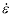Figure 62. Equation. Effective strain rate

Figure 63. Equation. Variation of fluidity parameter η in tension

Figure 64. Equation. Variation of fluidity parameter η in compression

Figure 65. Equation. Effective fluidity parameters, ηt, ηc, and η s

Figure 66. Equation. Overstress limit of η

Figure 67. Equation. Back stress α ij n + 1

Figure 68. Equation. Updated stress with hardening, σPij n+1

Figure 69. Equation. Incremental back stress, Δαij

Figure 70. Equation. Brittle rate of translation CH Brittle

Figure 71. Equation. Ductile rate of translation CH Ductile

Figure 72. Equation. The limiting function Gα.

Figure 73. Equation. Modified shear failure surface, Ff

Figure 74. Equation. Default Young's modulus E

Figure 75. Equation. Shear and bulk moduli, G and K

Figure 76. Equation. ACI Young's modulus, Ec

Figure 77. Equation. Reduced ACI Young's modulus, Ec

Figure 78. Equation. TXC Strength

Figure 79. Equation. Interpolation parameter P

Figure 80. Equation. Most general form for Q1, Q2

Figure 81. Graph. This isotropic compression simulation demonstrates how the cap parameters set the shape of the pressure-volumetric strain curve

Figure 82. Equation. The default fracture energy GF

Figure 83. Graph. Approximate tensile and compressive dynamic increase factors for default concrete model behavior

Figure 84. Illustration. General shape of the concrete model yield surface in two dimensions

Figure 85. Equation. Three stress invariants, J1, J2, J3

Figure 86. Equation. Plasticity yield function f

Figure 87. Equation. Shear surface function Ff

Figure 88. Equation. Most general form for scaling functions Q1, Q2

Figure 89. Equation. Cap surface function, Fc

Figure 90. Equation. Definition of L of kappa

Figure 91. Equation. Pressure invariant X as a function of kappa

Figure 92. Equation. Plastic volume strain hardening rule, ε pv

Figure 93. Equation. Transformation of viscoplastic stress to damaged stress, σdij.

Figure 94. Equation. Ductile damage accumulation, τ d

Figure 95. Equation. Brittle damage accumulation, τb

Figure 96. Equation. Brittle damage, d of tb

Figure 97. Equation. Ductile damage, d of τd

Figure 98. Equation. Reduction of A with confinement

Figure 99. Equation. Brittle and ductile damage thresholds, Gf

Figure 100. Equation. Viscoplastic stress, σvpij

Figure 101. Equation. Variation of the fluidity parameter η in tension and compression

Figure 102. Definition of effective strain rate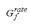Figure 103. Equation. Overstress limit of η

Figure 104. Equation. Fracture energy with rate effects

Figure 105. Computer printout. Example concrete model input for default material property input (option mat_CSCM_concrete)

Figure 106. Computer printout. Example concrete model input for user-specified material property input (option MAT_CSCM)

Figure 107. Graph. Example single element stress-strain results for 30 MPa (4,351 psi) concrete with 19-mm (0.75-inch) maximum aggregate size

Figure 108. Equation. Old generic damage, small d of τ

Figure 109. Equation. New generic damage, small d of τ

Figure 110. Graph. Behavior of the original softening function

Figure 111. Graph. Behavior of the updated softening function

Figure 112. Graph. Rebar yields in a ductile manner at a quasi-static rate of 0.0054/s. Source: U.S. Army Engineer Waterways Experiment Station.(29)

Figure 113. Graph. Rebar exhibits rate effects at a strain rate of 4/s. Source: U.S. Army Engineer Waterways Experiment Station.(29)

Figure 114. Equation. CEB tensile strength dynamic increase factor, DIFten

Figure 115. Equation. CEB compressive strength dynamic increase factor, DIFcomp

Figure 116. Graph. Dynamic increase factors specified in CEB

### List of Tables

Table 1. These default bulk and shear moduli of concrete are derived from the formula for Young's modulus provided in CEB.

Table 2. These bulk and shear moduli for concrete are derived from a formula for Young's modulus suggested by ACI Code Committee.

Table 3. Approximate strength measurements used to set default TXC yield surface parameters.

Table 4. TXC yield surface input parameters as a function of unconfined compression strength.

Table 5. Quadratic equation coefficients which set the default TXC, TOR, and TXE yield surface parameters as a function of unconfined compression strength.

Table 6. TOR yield surface input parameters as a function of unconfined compression strength.

Table 7. TXE yield surface input parameters as a function of unconfined compression strength.

Table 8. Cap shape, location, and hardening parameters as a function of unconfined compression strength.

Table 9. Coefficients for the fracture energy equation.

Table 10. Tensile fracture energies tabulated in CEB as a function of concrete strength.

Table 11. Example load curve for modeling rebar strain rate effects with LS-DYNA Material Model #24.

Glossary of Symbols
a a0 a1 a2
Rubin function internal parameters
A B C D
softening parameters (compression and tension)
AP  BP  CP
b b0 b1 b2
Rubin function internal parameters
Bs
term used in one rate effects formula
CH
hardening rate parameter
d d b d d
scalar damage parameter (general, brittle, ductile)
dm
maximum of brittle and ductile scalar damage parameters
dmax
maximum damage allowed to accumulate
D1 D2
cap linear and quadratic shape parameters

E EcEs
Young's modulus (general, concrete, steel)
f
yield surface function
f*
trial elastic yield surface function
Ff
shear failure surface
Fc
hardening cap surface
G
shear modulus
Gα
hardening model translational limit function for shear surface
Gft Gfc Gfs
fracture energies (tension, compression, shear)
J1
first invariant of the stress tensor
J ¢2 J ¢3
second and third invariants of the deviatoric stress tensor
J1T J ¢2T J ¢3T
trial elastic stress invariants
J1P J ¢2P J ¢3P
inviscid elastic stress invariants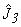normalized invariant of the deviatoric stress tensor
K
bulk modulus
L
element length
N
hardening initiation
nt nc
rate effects fluidity parameters (tension, compression)
Nt NC
rate effects power parameters (tension and compression)
P
pressure
Q1 Q2
Rubin scaling functions for torsion and triaxial extension
Rubin strength reduction factor
R
cap aspect ratio
rS
initial damage before activation of rate effects
r0 r0b r0d
initial damage threshold (general, brittle, ductile)
Sij
deviatoric stress tensor
W
maximum plastic volume compaction
x x0
instantaneous displacement and displacement at peak strength
X X0
current cap location and initial cap location
y
integrand of dilogarithm function
αij Δαij
hardening model back stress and incremental back stress tensors
β β1 α2
shear surface constant term (compression, torsion, extension)
βs
term used in one rate effects formula
β β1 β2
shear surface exponent (compression, torsion, extension)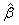angle in deviatoric plane (invariant)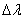plasticity consistency parameter
Δt
time step increment
εij Δ εij
strain tensor and strain increments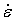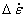effective strain rate and effective strain rate increment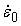term used in one rate effects equation
εmax
maximum principal strain
εx εy εz εxy εyz εxz
strain components
εv
volumetric strain
ε pv
plastic volumetric strain
γ
viscoplastic interpolation parameter
γ s
term used in one rate effects formula
ηηt ηcηs
rate effects fluidity parameters (general, tension, compression, shear)
η0t nt
rate effects input parameters in uniaxial tension stress
η0c nc
rate effects input parameters in uniaxial compressive stress
κ κT κP κ0
cap hardening parameters (general, trial elastic, inviscid, initial)
λ1 λ2
shear surface nonlinear term (compression, torsion, extension)
η
Poisson's ratio
θ θ1 θ2
shear surface linear term (compression, torsion, extension)
ρ ρc ρs
density (general, concrete, steel)
ρt ρc ρσ
meridians (tensile, compressive, shear)
σ σT
a stress component (general, trail elastic)
σ x σ r
axial and radial stresses measured in triaxial compression tests
σvp σd
stress components calculated without and with damage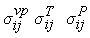stress tensors (viscoplastic, trial elastic, plastic)
σ 1 σ 2 σ 3
principal stress components
τbτd
instantaneous strain energy-type terms for damage accumulation
 ACI American Concrete Institute Comité Euro-Internacional du Béton continuous surface cap model dynamic increase factor Federation for Prestressing National Cooperative Highway Research Program torsion triaxial compression triaxial extension